A GENERAL TEST-BED FOR RECURSIVE SUBSTRUCTURING
IN STRUCTURAL DYNAMICS SIMULATION

BY
LLOYD VAN WARREN
B.S., University of Illinois, 1981

THESIS
Submitted in partial fulfillment of the requirements for
the degree of Master of Science in Aeronautical and Astronautical Engineering
in the Graduate College of the
University of Illinois at Urbana-Champaign, 1983

Urbana, Illinois

Lloyd Van Warren
1983

ACKNOWLEDGMENTS

I would like to first acknowledge the continual support and encouragement of my wife, Lynn Mittelstaedt-Warren. Also to be thanked is my advisor, Dr. Arthur L. Hale, who endured my ideas and absorption of finite element theory with patience. Thanks also goes to Dr. Harry H. Hilton, head of the department, for permitting me the opportunity to study aeronautical and astronautical engineering at the graduate level, and proceed headlong into computer science in the process.

My appreciation is also extended to Wayne "J.W." Hamilton and Patrick Kane who took me under their wings and enlightened me in the ways of UNIX. Also the kind cooperation of Debbie Hudson, Mike Randal, Larry Sautter, and George Badger of Computing Services Office, who enabled me to continue to have access to a wonderful programming environment for developing the programs described herein. Also due thanks are Turner Whitted and David Weimer, after whose 'Raster Graphics Software Test-bed' this 'Finite Element Test-bed' is patterned and who kindly provided me with high quality raster graphics software. The lectures and work of L. A. Lopez that helped me to understand the deeper software engineering problems in finite element analysis are also appreciated.

The financial support of the National Science Foundation via Grant No. DME-8105535 is also much appreciated.

 LIST OF TABLES *  LIST OF FIGURES *  CHAPTER 1 INTRODUCTION *  1.1 PRELIMINARY REMARKS *  1.2 SUBSTRUCTURING *  1.3 INTROSPECTION *  1.4 OBJECTIVES * CHAPTER 2 RECURSIVE SUBSTRUCTURING IN DYNAMIC ANALYSIS *  2.1 INTRODUCTION *  2.2 EIGENSOLUTION BY SUBSPACE ITERATION *  2.3 A RECURSIVE ASSEMBLY PROCEDURE WITH REDUCTION *  2.4 RECURSIVE SUBSPACE ITERATION *  2.5 DISTRIBUTED VS. CENTRALIZED SOLUTION CONTROL * CHAPTER 3 ATTRIBUTE MODELING *  3.1 INTRODUCTION *  3.2 OBJECT ATTRIBUTES *  3.3 ENVIRONMENT ATTRIBUTES *  3.4 ANALYSIS REQUESTS ATTRIBUTES * CHAPTER 4 DATA STRUCTURES DESIGN *  4.1 INTRODUCTION *  4.2 OBJECT DESCRIPTION * CHAPTER 5 DESIGN OF PROBLEM SPECIFICATION LANGUAGE *  5.1 INTRODUCTION *  5.2 GENERAL CONSIDERATIONS *  5.3 EVOLUTION OF GRAMMAR DESIGN *  5.4 OBJECT SPECIFICATION *  5.5 ENVIRONMENT SPECIFICATION *  5.6 ANALYSIS REQUESTS SPECIFICATION *  5.7 METHODOLOGY SPECIFICATION *  5.8 TOOLS FOR GRAMMAR IMPLEMENTATION * CHAPTER 6 ILLUSTRATIVE EXAMPLES *  CHAPTER 7 CONCLUSIONS *  BIBLIOGRAPHY *

 TABLE 2.1 SOLUTION METHODOLOGY STRATEGIES *  TABLE 4.1 RECURSIVE OBJECT DESCRIPTION FORMAT *  TABLE 4.2 TOPOLOGY DESCRIPTION FORMAT *  TABLE 4.3 NODAL DESCRIPTION FORMAT *  TABLE 4.4 NUMERICAL DESCRIPTION FORMAT *  TABLE 4.5 STATES DESCRIPTION FORMAT *  TABLE 4.6 MATERIALS DESCRIPTION FORMAT *  TABLE 5.1 CONSTRAINT COEFFICIENT MATRIX *  TABLE 5.2 METHODOLOGY ALTERNATIVES *

 FIGURE 2.1 THE METHODOLOGY TREE *  FIGURE 5.1 PROBLEM REPRESENTATIONS *  FIGURE 5.2 INPUT METHODS *  FIGURE 5.3 WIDGET *  FIGURE 5.4 FRAMISE *  FIGURE 5.5 BAR OBJECT *  FIGURE 5.6 PLATE OBJECT *  FIGURE 5.7 QUAD OBJECT *  FIGURE 5.8 LEXICAL ANALYSER - PARSER INPUTS & OUTPUTS *  FIGURE 6.1 THE 'PIECE' OBJECT *  FIGURE 6.2 THE 'PIECE2' OBJECT *  FIGURE 6.3 THE 'PIECE4' OBJECT *  FIGURE 6.4 THE 'NEWBAR10' OBJECT *  FIGURE 6.5 THE 'NEWBAR10' OBJECT FIRST NONZERO MODE *  FIGURE 6.6 THE 'NEWBAR10' OBJECT SECOND NONZERO MODE *  FIGURE 6.7 THE 'NEWBAR10' OBJECT THIRD NONZERO MODE *  FIGURE 6.8 THE 'BIGSQUARE' OBJECT *  FIGURE 6.9 THE 'BIGSQUARE' OBJECT FIRST NONZERO MODE *  FIGURE 6.10 THE 'BIGSQUARE' OBJECT SECOND NONZERO MODE *  FIGURE 6.11 THE 'BIGSQUARE' OBJECT THIRD NONZERO MODE *

CHAPTER 1
INTRODUCTION

1.1 PRELIMINARY REMARKS

Simulating the dynamic response of a complex structure to its environment requires the formulation of a suitable mathematical model of the structure in its environment and efficient solution algorithms for calculating the response. Also required are methods for specifying the problem in a convenient way, and methods for viewing the results.

The finite element method provides a universal tool for formulating mathematical models, as its pervasive use attests. The method is ideally suited to the digital computer, the mathematical model being formulated by a computer program. However, a complex problem's definition and its specification to a computer system can require considerable human effort. By modeling a complex structure as an assemblage of substructures, the human effort can be subdivided and the total effort reduced.

1.2 SUBSTRUCTURING

To distinguish between the concepts of data structures and engineering structures, the words 'object' and 'subobject' will be used to mean engineering structure and substructure respectively. The word 'object' is chosen to represent this general entity because it emphasizes the similarity between graphical and finite element representations. The words 'data structure' will continue to mean data structure, and the word 'substructuring' will be used as a verb, in place of the very awkward word 'subobjecting'.

Criteria for subdividing an object usually correspond to easily recognizable boundaries. The boundaries arise naturally during stages of design, fabrication and manufacturing. For a given object many possible divisions into subobjects exist. Moreover, the division can have many levels. If, at the most fundamental modeling level, finite elements are considered as subobjects, then a subobject at any higher level can be specified as a collection of already defined subobjects. The result is a natural building block approach to problem specification. The model for each subobject, starting at the lowest level, can be formulated from its specifications and ultimately a full problem model can be obtained.

Note that two views of substructuring are defined, namely, 1) the process of subdividing from the top down and 2) the process of successive specification from the bottom up. Because both views are useful, it is important that the specification process not be limited to one view or the other.

Once a problem is completely specified, the response is obtained; hopefully, it is obtained by an efficient solution algorithm. A full finite element model for a complex object typically possesses a large number of degrees of freedom. The number often exceeds ten or even one hundred thousand. The large number results from the finite element modeling process and not from the complexity of the expected response. Therefore, a computational model possessing a substantially reduced number of degrees of freedom is desirable, especially if computational economy can be gained. To this end, substructuring, possibly multileveled can be exploited. The idea is almost twenty years old for both linear statics [PRZE63]and linear dynamics analyses [HURT65]. In this context, substructuring is now part of a solution algorithm. The accepted practice is to use the same division into subobjects as that chosen for problem specification. However, a different division may be more appropriate for a given task..

Substructuring concepts for static analyses, including computer software implementation, are at a high level of development [NOOR78, PETE77, DODD80].One reason is perhaps the simplicity of static analyses. Each subobject model can be exactly reduced to its boundary degrees of freedom by static condensation. Then, statically condensed subobjects can be assembled to form the whole object model. For linear problems, any computational economy gained depends on the choice of subobjects and on how many identical subobjects are involved [NOOR78].Even if little or no economy is gained, an advantage of substructuring in linear static analyses is simplified problem specification. The properties of good finite element software for implementing substructuring and easy problem specification are discussed in [SCHR79, LOPE82, DODD82].

Exact condensation of a subobject model to its boundary degrees of freedom cannot be realized in the time domain for dynamic analyses. An exact condensation can be performed in the frequency domain although frequency dependent subobject coefficient matrices are introduced [BERM73]. Because constant coefficient matrices are desirable, many approximate methods for reducing the number of subobject degrees of freedom in the time domain have been proposed. One alternative is to ignore the time dependence of the internal degrees of freedom and use static condensation. Another possibility is to represent the response of each subobject by a combination of its lower modes of vibration. The latter technique is known as component modes synthesis [HURT65, DOWE72, BENF71, MACN71, HINT75, CRAI77]. Component modes synthesis is a special case of the general substructure synthesis method [HALE80, MEIR81].The general substructure synthesis method is a Rayleigh-Ritz method, wherein the order of each subobject is reduced before the subobjects are coupled together to form the whole object. Finally, novel algorithms for iteratively improving the reduced representation of each subobject in the object synthesis have been proposed [HALE82a, HALE82b].

1.3 INTROSPECTION

This thesis builds on the general substructuring algorithms of [HALE82a, HALE82b] for dynamic analyses by considering the "software engineering" concepts required to implement the general substructuring algorithms. The premise is that algorithms and their associated software implementation must be considered together if the algorithms are to become useful tools. The reader familiar with computer graphics will observe that the present test-bed for recursive substructuring follows pattern set in [WHIT81].

Development of substructuring software is easiest in a proper computational environment [LOPE82, DODD82]. The first requirement is a high level structured programming language that has general data structuring capability so that problem attributes can be divided into convenient conceptual groups for easy referencing by the programmer. A second requirement is a language that allows pointers to data to eliminate unnecessary copying and moving of large amounts of data. A language/operating system environment that permits dynamic allocation of memory also helps to provide for efficient use of resources when problem solution is underway.

Because the test-bed runs in a virtual memory environment, there is not yet much concern about how large problems are. There is interest in only using as much memory resource as is required for the current state of the problem. A third requirement is a software environment that possesses a good human interface, and good software building and maintenance tools.

A data structure is the computational embodiment of any representation scheme. For recursive substructuring it is important that all object data structures look the same whether a simple or a complex object is being modeled. When object data structures have the same appearance, fewer software modules are required to solve the problem, provided the special case at the top or the bottom level of object definition is eliminated. The price paid for this generality in the present system is negligible. In fact, it forces the problem to be posed in a self-consistent way that is independent of level.

Objects also have the same set of attributes whether they are complex or simple. Little distinction is made in the test-bed between a bar element and a complex structure with a thousand degrees of freedom. This is accomplished economically through the use of data structures which contain pointers to records, rather than the records themselves. For example, both a bar element and a bridge possess the attributes of topology and material constitution. But, a bridge is more complex topologically and materially than a bar. In other words, it is the specific instances of the attributes that differ in complexity. A more important difference is the fact that a bar has no subobjects, while a bridge does. This provides a recursion termination criterion. If an object has no subobjects, then it is an element and its mass and stiffness matrices either already exist, or they can be found with a single procedure call.

The fact that the same data structure is used to represent objects regardless of the substructuring level is important because it allows programs to be ignorant of the complexity of the entity upon which they are currently operating. Using the same data structure at every level also has some nice implications when writing routines that exploit the recursive nature of the object description. There are two primary types of procedures that occur in the test-bed, namely, those that operate on several kinds of object data simultaneously, and those that manipulate only one kind of data. The latter tend to be utilities such as a matrix multiplication procedure. Of the former procedures, several important software modules in the test-bed are recursive and they require access to many kinds of object data. A typical recursive module takes a single argument which is a pointer to the data structure for the object it is currently processing. The ease with which passing pointers allows access to all of an object's data cannot be overemphasized.

Next, the test-bed user can specify problems via a problem specification language that is similar in its syntax to the Lisp language. Using a Lisp-like syntax has a couple of advantages. First, it eliminates ambiguities in the grammar of the problem specification language. Second, it allows an easy transition from the present test-bed to one that is written in Lisp. The power of Lisp as a language for problem specification and for computation is skillfully discussed in [REYN78, REYN82]. In his Actor/Scriptor Animation System (ASAS) Reynold's goes at least one order of magnitude past current computer graphics systems is his ability to define complex objects, and to define and manipulate geometric and photometric relationships between objects.

Finite element analysis and computer graphics share several important characteristics. They are both areas which tend to require the user to express complex topologies and object relationships. Both also attempt to model complementary segments of the real world and both activities tend to be compute bound. In computer graphics, the expense is in generation of shaded three-dimensional images for animated scenes, while in finite element analysis the expense is in the calculation of an object's response history.

1.4 OBJECTIVES

The goal of this work was to build a finite element test-bed for the evaluation of substructuring and order reduction algorithms. The goal of these techniques is reducing the cost of finding the response of an object to its environment. An easily reconfigurable test-bed has been constructed that allows many new and novel algorithms to be tested in a straightforward fashion.

CHAPTER 2
RECURSIVE SUBSTRUCTURING IN DYNAMIC ANALYSIS
2.1 INTRODUCTION

A recursive procedure is defined for purposes here as a procedure that can repeat itself until a specified condition is met. If a given object is considered to be made of subobjects which are themselves made of subsubobjects, etc, then object specification is recursive. Herein, the recursion terminates when an object's data is defined only via a procedure call. The procedure call is usually associated with a basic finite element. However, the test-bed does not require the object to be a basic finite element and very complex procedures can be invoked. Recursive substructuring can be further justified by noting a number of popular algorithms in numerical analysis and computer graphics that also lend themselves well to recursion. Polynomial root finding, adaptive quadrature for numerical integration, Warnock's hidden line algorithm which recursively divides and conquers, and Catmull's recursive bicubic patch subdivision are four examples [COHE82].

Making objects' definitions similar at every level has some very desirable consequences, both for the user defining the problem and for the software modules processing the problem. This idea is not new to finite element programs; the POLO FINITE static analysis program [DODD80] is an example. First, from a software point of view, a given module does not need to know explicitly all of an object's complexity or at what level in the object definition it operates. A software module or 'processor' can be given the data for an object and then call itself when appropriate for each subobject of that object. Second, from a problem input point of view an object is defined once. The defined object can be used as a subobject of another object and it can be used at any level.

This 'building block' process can be very powerful. It allows the user to rapidly specify complicated topologies with little effort. In addition, work done one day defining objects is useful on another when the same object is needed again.

Because this thesis emphasizes the test-bed itself rather than the large variety of substructuring computational algorithms that it can implement, this chapter is intended to be only illustrative of recursive substructuring. For simplicity, the eigenvalue problem for undamped free vibration is chosen along with the subspace iteration method for obtaining the eigensolution.

2.2 EIGENSOLUTION BY SUBSPACE ITERATION

The natural frequencies and natural modes of vibration for a complex object are obtained by solving the eigenproblem:(2.1)

where: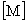and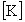are the N by N symmetric mass and stiffness matrices of the object,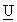is the N dimensional displacement vector and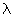is an eigenvalue.

For objects with a large number of degrees of freedom, the response usually involves the first few modes only. Rather than solve the full order eigenproblem for all modes, it is necessary to obtain only the lower modes. The mass and stiffness matrices can be reduced, perhaps by several orders of magnitude, via the Rayleigh-Ritz method and the reduced eigennproblem solved. The resulting eigensolution only approximates the actual lower modes. The accuracy of the approximation can by increased by generating improved Ritz trial vectors and repeating the reduction process iteratively. This procedure is known as 'subspace iteration' and is described along with several of its novel variants in [HALE82b]. Other references for the classical subspace iteration method are [PARL80, BATH82, JENN77, MEIR80].

The classical subspace iteration method is described by the following steps. In the following development please note that the superscript does not imply exponentiation, but rather an iteration number.

1) Rayleigh-Ritz Step

a) Represent an eigenvector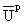at iteration p as the sum: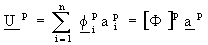(2.2)
of n independent trial vectorsand form the n dimensional reduced eigenvalue problem: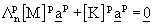(2.3)

b) Solve (2.3) for the n computed eigenvaluesand the associated eigenvectors(r = 1, 2, ..., n).

c) Compute the associated approximate eigenvectors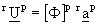(2.4)
2) The Subspace Improvement Step a) Generate n improved trial vectors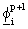by solving(2.5)
Next, it is informative to consider a choice of Rayleigh-Ritz trial vectors in conjunction with a modification to the above algorithm. The motivation is the theme of treating objects on all levels in the same way. Therefore, the object considered above should be treated in the same way as an object dO at recursion depth d. Consider an object at recursion depth d, denoted by dO, to be divided in some convenient way into m subobjects doi, i = 1, 2, ..., m. It is understood that each subobject can be further subdivided, and the process continues until the recursion terminates.

Each subobject doi acts as part of the parent object dO and the eigenproblem for dO is described within doi by the equations: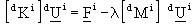(2.6)

where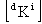and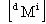are the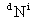bysymmetric mass and stiffness matrices,is thedimensional displacement vector andis an eigenvalue. During the assembly process only those degrees of freedom associated with the boundary of the object are transformed. The eigenproblem at iteration p for the object doi at recursion depth d is posed in terms of the following partitioned matrix equations [HALE82b]:(2.7)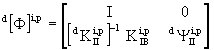(2.8)

The improvement process is then given by: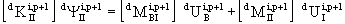(2.9)

For considerably more detail see [HALE82b].

2.3 A RECURSIVE ASSEMBLY PROCEDURE WITH REDUCTION

All finite element programs exploit the ease with which complex mass and stiffness models can be built by assembling the submatrices together into the matrix for the parent object. Because the assembly process is well known and is inherently hierarchical, see [BATH82, COOK81, MEIR80, ZIEN77], it is a good example of a recursive procedure. Explicitly, recursive assembly can be described by the following general procedure:

procedure assemble (A: Object A)
begin for (each subobject ai in A) do
begin if (ai mass and stiffness 'formed state' is NO)
begin assemble(ai) end
end

if (A mass and stiffness 'formed state' is NO)
begin

get space for mass, stiffness, and right hand side end

if (A is an element)
begin

if (A mass and stiffness 'formed state' is NO) call A's element procedure end
else
begin for (each subobject ai in A) do
begin if (ai is an element) call element procedure for ai put ai mass, stiffness, and right hand side into A's end
end
set A 'formed state' to YES
if (A has more than permitted internal degrees of freedom)
begin get space for reduced mass, stiffness, and right hand side
form the desired number of Rayleigh-Ritz basis vectors for reduction
reduce A's mass, stiffness right hand side
set A reduction state to YES
end
end

This assembly procedure starts with the parent object and continues until all the children on all levels are processed. It is important to note the appearance of the Rayleigh-Ritz reduction process in the bottom if clause of procedure assemble. When reduction is incorporated into the assembly process, the number of degrees of freedom of objects on any level can be regulated uniformly and automatically. The same assembly procedure is used whether reduction of an object is desired or not. If reduction is not desired, the number of permitted internal degrees of freedom need only be specified as a number larger than the number of degrees of freedom in the object.

2.4 RECURSIVE SUBSPACE ITERATION

When the concept of subspace iteration is combined with recursive assembly and reduction on several levels of a problem, a host of possible multi-level iterative algorithms arise [HALE82b]. Note that in the following discussion, function returns a value while a procedure does not.

One procedure for 'multi-Ritz' is given below:

assemble(A)

procedure multi_Ritz (A: Object A)
begin

while (converged is NO)
begin solve eigenproblem for A
converged = recur_iterate(A)
end
end

The function for 'recur_iterate' is then:

function recur_iterate (A: Object A)
begin

if(object A's mass and stiffness 'reduction' state is YES)
begin if(object A's eigenproblem not converged)
begin expand A's reduction basis vectors
improve A's reduction basis vectors
reduce A's mass, stiffness and right hand side using basis vectors
return(NO)
end
end
if(reduction occurs below A)
begin if(A is reduced)
begin expand A's reduction basis vectors end
for (each subobject ai in object A) do
begin if (ai is reduced or has reduced subobjects)
begin
pass A's eigenvectors downward to ai
a_yes_no = recur_iterate(ai)
if(a_yes_no is NO)
begin
A_yes_no = NO
end
end
if(A_yes_no is NO)
begin reassemble(A)
return(NO)
end
end
return(YES)
end

The procedure reassemble is similar to assemble and is omitted for brevity.

2.5 DISTRIBUTED VS. CENTRALIZED SOLUTION CONTROL

There are many alternatives to monitoring and controlling the flow of the solution in a dynamics problem analysis. The phrase 'solution control' means the choice of methodologies or procedures to invoke when the problem is in a state where there is more than one way to proceed to a solution. Optimally, the path through the methodology options tree that minimizes the cost of solution is desired. Finding the optimal solution path is beyond the scope of this work. It is nevertheless an interesting problem and it represents the 'right' approach to 'solution control'.

Given a set of basic procedures that can be used to perform an engineering analysis, there are several alternatives. The basic procedures might include assembly, reduction, constraint imposition, etc.. The procedures can be viewed as operators in the mathematical sense.

Presently, a distributed solution controller implemented via a set of solution state flags is used. Each procedures looks at the object state flags while the problem is being solved and makes appropriate decisions. The optimum methodology should be chosen on the basis of minimum cost. If estimating the cost of subproblem solution is expensive with respect to solving the subproblem this fact should be returned to the calling procedure which could then make a decision about whether to go ahead and solve the subproblem. This amounts to estimating the cost of estimating the cost. Some options that can be chosen for three typical finite element operators are listed in Table 2.1.

 TABLE 2.1 SOLUTION METHODOLOGY STRATEGIES recursive operator possible main strategy possible sub-strategy assemble all at once  on the fly breadth first  depth first  breadth first  depth first reduce all at once  on the fly breadth first  depth first  breadth first  depth first constrain all at once  on the fly breadth first  depth first  breadth first  depth first

There are a large number of solution paths. The options that occur at a given step in the solution comprise a set of possible branches which define a methodology tree (see figure 2.1).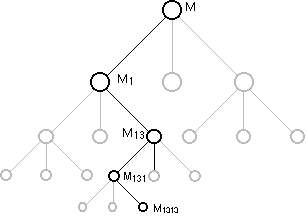FIGURE 2.1 THE METHODOLOGY TREE

Good controllers should attempt to reach the bottom of the tree via the minimum cost path. It can be difficult and expensive to find this minimum cost path; it may often be more expensive than solving the problem! Therefore, heuristics can be used that make a good decision based on available information. In the present setting, heuristics can be used to toggle state flags that will determine the solution path. This idea of using heuristics has been implemented only to a primitive degree in the current test-bed.

CHAPTER 3
ATTRIBUTE MODELING

3.1 INTRODUCTION

Engineering problems have attributes that can be drawn from four basic categories, namely, object attributes, environment attributes, analysis requests attributes (what answers does the user want), and methodology attributes (how the user wants those answers to be obtained). The goal of an analysis and its associated bookkeeping must be separated from the mathematical model of the problem.

3.2 OBJECT ATTRIBUTES

A generalized object is a computational entity comprised of zero or more subobjects. To construct an attribute model one must answer a series of questions. For example, "What are the subsets that describe the set of current interest?". Also, "What are the attributes associated with each subset?". Finally, "What are the states associated with each of the attributes?". Because attribute modeling is a procedure, it could be automated. Since it can be automated, it is possible that a machine could do the attribute modeling. If the relationships between the attributes can be extracted by a similar set o questions, then that too could be automated. Simulating the conversation between a person and a machine enables the attributes to be extracted in an orderly and self-consistent fashion.

The conversation might proceed as follows:

 Machine: "What are the attributes of an object?" Person: "An object has:          identification information,          topology,          materials,          nodes,          subobjects,          an object mathematical model." Machine: "What are the attributes of identification information?" Person: "Identification information has:      a name,      a number." Machine: "What are the attributes of topology?" Person: "Topology has:          internal topology,          external topology." Machine: "What are the attributes of internal topology?" Person: "Internal topology has:          a list of relationships between subobjects." Machine: "What are the attributes of external topology?" Person: "External topology has:  a list of relationships between the current object and other objects." Machine: "What are the attributes of materials?" Person: "Materials have:          identification information, (machine has seen this above!)          a material mathematical model." Machine: "What are the attributes of nodes?" Person: "A node has:          identification information, (machine has seen this above!)          physical coordinates,          degrees of freedom." Machine: "What are the attributes of subobjects?" Person: "A subobject has:          all the attributes of an object" (recursive definition) Machine: "What are the attributes of an object's mathematical model?" Person: "An object mathematical model has:          mass matrices,          damping matrices,          stiffness matrices,          relational or boolean matrices,          element procedures,          force vectors,          displacement vectors,          velocity vectors,          acceleration vectors,          stress vectors."

The conversation is continued until the questions all result in a primitive data type, such as a number or a character string. Associated with each attribute are states of interest that could be derived from a similar conversation. States include whether an attribute is unique or shared among several objects and whether an attribute is defined.

3.3 ENVIRONMENT ATTRIBUTES

In addition to the description of the object itself, in finite element analyses objects are placed in and interact with environments. Often there is ambiguity whether certain quantities such as forces are attributes of the object or of the environment. Because forces are associated with specific regions of the object, they could be associated with the object. But outside forces are really just external signals coming from the environment. Therefore, it seems that they should be an environmental attribute rather than an object attribute. This paradox surfaces often when one considers the 'who forces who' question. The domain of the object upon which the force acts is definitely an attribute of the object. The force itself, however, belongs to the external environment.

An environment can have:
a field, e.g. temperature or body force field,
a set of forces which impose themselves on the object at
specific locations.

3.4 ANALYSIS REQUESTS ATTRIBUTES

Finally, it is necessary to configure the analysis procedures so that requested answers are provided, hopefully with the minimum utilization of resources.

Analysis requests have:
unknown quantities (e.g. stresses, displacements or vibrational modes)
computational costs.

Because of the programming difficulty, this has computational cost analysis has not been implemented.

CHAPTER 4
DATA STRUCTURES DESIGN

4.1 INTRODUCTION

Objects in environments are the what and where of engineering problems. By listing the attributes of objects and environments, one represents these problem entities in an orderly fashion. The attributes must also be represented by the machine in an orderly fashion, where the machine representation consists of a data structure.

In designing finite element software, especially easily reconfigurable codes, it is desirable to structure the problem's data in a logical and self-consistent way. By using a structured programming language that supports data structuring, it is only necessary to define the data structures that group the problem attributes in a way suitable for efficient storage, solution and coding.

Efficient storage is gained by aligning frequently referenced quantities. This is somewhat machine dependent. As far as the data structuring goes, efficient manipulation is obtained by minimizing the number of pointer calculations that are necessary to reference a value. Deeply nested data structures are to be avoided for frequently referenced items. Efficient coding is allowed when related data items are clustered in a natural way.

When designing data structures, the difficult question of whether to use the hierarchical, relational, or network approach to organizing the data arises. The details of each approach to data management I well covered in [DATE76]. In chapter 3 of [DATE 76] on data structures and operators it is stated that "... hierarchies are obviously a natural way to model truly hierarchical structures from the real world". This is the approach taken herein. Nevertheless, the test-bed data structures can be cyclically linked to resemble the network approach if need should arise. The problem with the hierarchical approach is that it is possible to ask questions about an object that require a search of all the branches in the hierarchy. For instance, if one wants to know which nodes of the root object correspond to the nodes of a bottom level object, one has to search and correlate. However, for complex objects the cost of traversing the tree is small when compared to the cost of a complicated problem's solution.

4.2 OBJECT DESCRIPTION

Because an object description must be compact, pointers to records are always used at the object description level in lieu of the records themselves. This has some nice implications when movement of data becomes necessary. Pointers can be copied or reassigned rather than large records. In the complete description of an object, a rather complex pointer network is created.

In table 4.1 the attributes of an object and pointers to instances of each attribute are recorded. Note that only pointers occur in the object description. This allows for compactness and for ease of generalizing the data structures while minimizing the propagation of changes to test-bed procedures.

 TABLE 4.1 RECURSIVE OBJECT DESCRIPTION FORMAT ATTRIBUTE INSTANCED BY identification pointer to i.d. description topology pointer to topology description materials pointer to materials description numerical information pointer to numerical description states pointer to states description environment pointer to environment description

Table 4.2 contains the topological or connectivity information for the object. Internal topology is recorded via the subobject list. External topology, i.e. what objects neighbor this object, is recorded via the incidence table.

 TABLE 4.2 TOPOLOGY DESCRIPTION FORMAT ATTRIBUTE INSTANCED BY nodes a) number of nodes  b) pointer to list of pointers to node descriptions  c) list of boundary nodes subobjects a) pointer to list of pointers to subobject's object descriptions b) number of subobjects incidences pointer to incidence table nodal reordering permutation vectors

Table 4.3 represents the recorded attributes of a node.

 TABLE 4.3 NODAL DESCRIPTION FORMAT ATTRIBUTE INSTANCED BY geometric coordinates x1, x2, x3 normal direction nx1, nx2, nx3 constraint state pointer to constraints degrees of freedom pointer to vector containing displacements, stresses, etc.

The mathematical model for the object is contained in the numerical data structure (table 4.4) which contains pointers to arrays of all pertinent numerical information. Using several levels of pointers need not be slow for numerical computations, because once a record is located, a single level pointer to that record can be used to access and assign its contents. Test-bed routines that deal with matrices utilize this and tend to be quite reasonable in their execution speed.

 TABLE 4.4 NUMERICAL DESCRIPTION FORMAT ATTRIBUTE INSTANCED BY full mass matrix pointer tofull stiffness matrix pointer to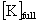full right hand side vector pointer to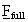reduced mass matrix pointer to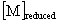reduced stiffness matrix pointer toreduced right hand side vector pointer toeigenvalues vector pointer to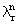full eigenvectors matrix pointer toreduced eigenvectors matrix pointer to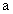basis vectors pointer to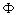constraint matrices pointer to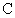element function pointer pointer to element subroutine numerical states list of state flags

The states description (table 4.5) is used to characterize the object's state at a given time during the computational procedure. This permits the use of adaptive algorithms.

 TABLE 4.5 STATES DESCRIPTION FORMAT FLAG PURPOSE def_by_fun indicates if object is an element formed indicates if object has data linear indicates if object is linear symmetric indicates if object has symmetric formulation reduced indicates if object has been reduced reordered indicates if object have been reordered num_replicate indicates if object is a numerical replicate of another num_times indicates number of occurrences of object at this level

The materials description (table 4.6) holds the object's material properties, and provides a slot for simple or complex material models.

 TABLE 4.6 MATERIALS DESCRIPTION FORMAT ATTRIBUTE INSTANCED BY elastic modulusmass density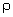The present approach has aptly been called 'pointers gone wild', particularly because of the tracking through 3 or 4 nested levels of pointers to pointers to pointers. The programming problem becomes on of managing the pointer network. This can be done by associating states with each pointer in the net. If a pointer actually points to something, i.e. space has been allocated, then a bit can be set to indicate that it is permissible to access the record. This idea leaves the job of checking and allocating space to active procedures. The overhead of toggling the bit is quite small. Moreover, the idea extends easily to the management of sparse and full matrices that are stored in one dimensional form. Two or three bits are sufficient to know the allocation state and the storage type for an array.

CHAPTER 5
DESIGN OF PROBLEM SPECIFICATION LANGUAGE

5.1 INTRODUCTION

There are two kinds of problem representation that are of concern: the first is the external human representation, and the second is the internal machine representation. A parser is required for proper translation of an external language representation to an internal one.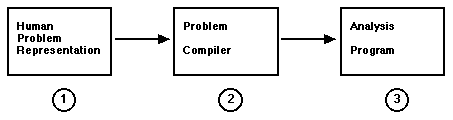FIGURE 5.1 PROBLEM REPRESENTATIONS

These human and machine problem representations can be expanded further:

A) Human Problem Representation

1) Pictorial Representation

2) Alphanumeric Representation

B) Machine Problem Representation

1) High Level Programming Language Rep

2) Lower Level Programming Language Rep

n) Binary Machine Language Rep

A good interface should allow interactive specification of the engineering problem at hand via a graphic medium. The output of such an interface would be a problem definition that could then be used by the parsing program to generate the data required for solution of the problem.

This chapter discusses the design and implementation of a problem specification language (PSL) that is modular, easy to use, compact and self-consistent. Until an interactive front-end for graphic problem specifications is written, an alphanumeric language will suffice. The language can later serve as a guide for an interactive and graphic problem specification program (see Figure 5.1).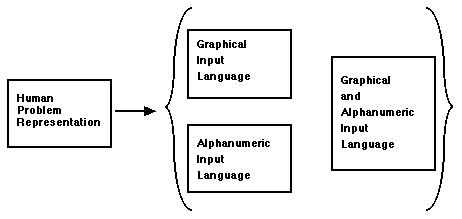FIGURE 5.2 INPUT METHODS

The test-bed contains a problem parser that translates a language specification into a binary form that is processed by the analysis program. This is adequate for testing algorithms, but could be improved so that a recompile would not be necessary to try out a new solution algorithm.

The goal is to provide an easily altered form of specifying problems. Previous efforts in this area are described in [DODD80]. The present goals are modularity and reasonable speed. The pitfalls of monolithic software systems, that are avoided here are discussed in detail by Schrem [SCHR79] and Felippa [FELI81].

5.2 GENERAL CONSIDERATIONS

Each problem requires a set of description statements that are collected and parsed together. Object A is made of subobjects a, b, and c, which are in turn made of subobjects, etc. Therefore, the specification of complicated objects is hierarchical. Each object has a set of nodal coordinates which represent a local coordinate basis. The local coordinates can be transformed into global coordinates automatically when the object is used as a subobject of another object. This way trouble doesn't arise when an object has subobjects which are all replicates of each other. Complete global coordinate information for each node, when it is needed, can be generated from coordinate transformations. The global coordinate system is just the local coordinate system for the object that is currently under analysis. This suggests that it may be appropriate to solve the subobject problems in their own coordinate systems and then transform the results only for those control points (usually nodes) which are common both to the subobject and object itself.

There are two main approaches to problem specification that must be permitted. In the first approach, a problem is defined by building up from available library objects. Library objects can be elemental objects or objects of arbitrary complexity defined in previous sessions. This is a powerful 'bottom up' approach. In the second approach, a complex problem is presented and it is desired to develop an accurate finite element model. This approach is top down in that one starts with a complete problem definition.

Also to be given attention in the design of a PSL is the fact that while an object can be very complicated internally, it often is connected to the outside world at relatively few points. A PSL should exploit this fact and allow the connectivity information to be expressed in terms of this fewer number of control points.

5.3 EVOLUTION OF GRAMMAR DESIGN

Several evolutionary stages took place in designing the grammar for the test-bed. In the first stage, specific instances of objects and environments were listed in tables, where each table represented some attribute. In the second, delimiters were introduced so that the problem specification could be context free. In the third stage, the delimiters were restricted to open and closing parentheses, and a nested list form was imposed. The final result is a Lisp-like input grammar [WINS81].

It is interesting to explicitly discuss the evolution of the grammar. First, the nodal coordinates a of a quad element were presented in a table as follows:

 Node x y z 1 0.0 0.0 0.0 2 1.0 0.0 0.0 3 1.0 1.0 0.0 4 0.0 0.0 0.0

This simple table illustrates the presense of some important descriptive issues. The keyword 'NODES' is an attribute label. The next line 'node x y z' is a header that establishes the format of what follows. The header is followed by the entries themselves. In this first stage, the white space surrounding the data was significant, i.e. formated input was used.

In the second incarnation of the grammar, a context free specification was sought that did not depend on the concept of a line, or on the precise nature of white space that surrounds the characters. In order to obtain an unambiguous grammar, delimiters were introduced. The tables took the form of chunks of programming language code. The above table was transformed to the following:

 { NODES 4          node x y z ;          1 0.0 0.0 0.0 ;          2 1.0 0.0 0.0 ;          3 1.0 1.0 0.0 ;          4 0.0 0.0 0.0 ;  }
The general format is expressed as follows:

 { ATTRIBUTE_KEYWORD number          header ;          data record 1 ;          data record 2 ;          ' ' ' ;          data record n ;  }

The presense of the header is interesting because it allows the program that parses the chunk to be 'data driven' (see [WINS81]). The program is expected to look at the header, and then decide what should be done with that data. This allows building some intelligence into the program in a clean and modular way. A popular example is a program that calculates the area of a geometric figure. The header would state whether the figure is a square or a circle or a polygon.

After reflecting on the second version of the grammar, it became clear that the attribute keyword could be viewed as an operator. Because a more general grammar than the above 'C' syntax was needed, the grammar was converted to a Lisp-like syntax. The advantage of Lisp is that, in Lisp, programs and data have the same appearance. Lisp can also provide a consistent framework for problem specification and solution regardless of how complicated the problem becomes. This is because Lisp is self-consistent and possesses a sound mathematical basis [CHUR41]. In attempting to specify problems at this level, natural language input or a more syntactically rigorous variant of natural language was the goal. Terry Winograd's pioneering work in natural language understanding [WINO74] discusses a Lisp based attack of this problem. Recent examples of the expressive power of Lisp based systems are the Animated Script and Actors system by Craig Reynolds [REYN78, REYN82]. Finite element analysis possesses enough complexity that the capabilities of Lisp become indispensable if progress is to continue in reasonable time. The Lisp-like version of the table is:

( NODES 4
( node x y z )
(
( 1 0.0 0.0 0.0 )
( 2 1.0 0.0 0.0 )
( 3 1.0 1.0 0.0 )
( 4 0.0 0.0 0.0 )
)
)

where the general format of the table is:

( ATTRIBUTE_KEYWORD number
(
( data record 1 )
( data record 2 )
( ' ' ' )
( data record n )
)
)

The tables are now Lisp expressions with two arguments which are also lists. The first list is a header and the second is the data. The current test-bed uses this syntax. Future versions could seek to view the attribute keyword as an operator that associates a name with a specific instance of data of that type. The act of associating a list with a name is done in Lisp with the setq operator. Moreover, the attribute keyword and header serve to provide type information. The above list is of type keyword (e.g. of type NODE in this example). A keyword type list has list structure given by the header (e.g. 'node x y z'). It may well be that the two concepts should be separated. The function of the headers could be stored elsewhere in a list of templates that define the list structure. The separation could save space when specifying a problem, although it is nice to attach the header to the list so that the structure is known without a search. The resulting syntax would look like:

( NODES framise_nodes
( node x y z )
(
( 1 0.0 0.0 0.0 )
( 2 1.0 0.0 0.0 )
( 3 1.0 1.0 0.0 )
( 4 0.0 0.0 0.0 )
)
)

Note that use of names as references makes it easy to refer to an instance of an object without needless repetition. A name can also be a number when alphameric naming would be to clumsy.

5.4 OBJECT SPECIFICATION

Problem specification consists of a list of tables. The hypothetical object, illustrated in figure 5.3, called a widget serves as an example. In a problem using the widget, the following object specification could be used:FIGURE 5.3 WIDGET
( OBJECT widget
( TOPOLOGY
( SUBOBJECTS 3
( subobj name )
(
( 1 framise )
( 2 framise )
( 3 framise )
)
)
( NODES 3
( node x y z )
(
( 1 0.00 0.00 0.00 )
( 2 0.50 1.00 0.00 )
( 3 1.00 0.00 0.00 )
)
)
( INCIDENCES 3)
( subobj incidences )
(
( 1 1 2 )
( 2 2 3 )
( 3 3 1 )
)
)
)
)

Notice that the widget is made of a triangular ring of three framises. Each framise appears to have two nodes, where the apparent nodes have the coordinates listed. The description of the framise (figure 5.4) is given below:

( OBJECT framise
( MATERIALS
( E rho )
( 1.0 1.0 )
)
( TOPOLOGY
( SUBOBJECTS 5
( subobj name )
(
( 1 beam )
( 2 beam )
( 3 plate )
( 4 beam )
( 5 beam )
)
)

( NODES 5
( node x y z )
(
( 1 0.00 0.00 0.00 )
( 2 1.00 0.00 0.00 )
( 3 0.25 0.25 0.00 )
( 4 0.75 0.25 0.00 )
( 5 0.25 -0.25 0.00 )
( 6 0.75 -0.25 0.00 )
)
)

( INCIDENCES 5
( subobj incidences )
(
(1 1 3 )
(2 1 5 )
(3 3 4 5 6 )
(4 4 2 )
(5 6 2 )
)
)

( BND_NODES 2
(1 2)
)
)
)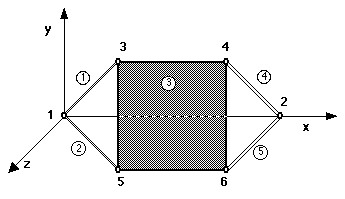FIGURE 5.4 FRAMISE

Note that a framise actually has 6 nodes, but the first two nodes correspond to the references made in the widget topology description and they are specified as boundary nodes. Also the nodal coordinates are listed with respect to a local coordinate system. Finally note the reference to an object (the bar in this case) that has no subobjects. Indeed, the bar is considered a finite element (see figure 5.5).

The bar topology specification is: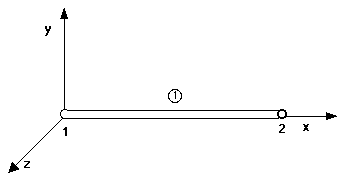FIGURE 5.5 BAR OBJECT

( OBJECT bar
( TOPOLOGY
( NODES 2
( node x y z )
(
( 1 0.00 0.00 0.00 )
( 2 0.00 1.00 0.00 )
)
)
)
)

A plate is also listed as a subobject of the framise. The plate (see Figure 5.6) is comprised of four quad objects and it is specified as follows: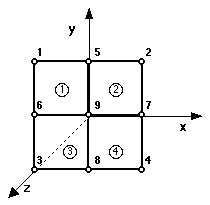FIGURE 5.6 PLATE OBJECT

( OBJECT plate
( TOPOLOGY
( SUBOBJECTS 4
( subobj name )
(
)
)
( NODES 9
( node x y z )
(
( 1 -1.00 1.00 0.00 )
( 2 1.00 1.00 0.00 )
( 3 -1.00 -1.00 0.00 )
( 4 1.00 -1.00 0.00 )
( 5 0.00 1.00 0.00 )
( 6 -1.00 0.00 0.00 )
( 7 1.00 0.00 0.00 )
( 8 0.00 -1.00 0.00 )
( 9 0.00 0.00 0.00 )
)
)

( INCIDENCES 4
( subobj incidences )
(
( 1 1 5 6 9 )
( 2 5 2 9 7 )
( 3 6 9 3 8 )
( 4 9 7 8 4 )
)
)
)
)

The quad object topology specification completes the specification of the example object. The quad (see Fig 5.7) has no subobjects and is therefore a finite element. The quad's topology follows: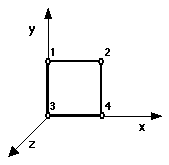( TOPOLOGY
( NODES 4
( node x y z )
(
( 1 0.00 1.00 0.00 )
( 2 1.00 1.00 0.00 )
( 3 0.00 0.00 0.00 )
( 4 1.00 0.00 0.00 )
)
)
)
)

An advantage of the above method of topology specification is that subobjects can be resized or rescaled simply by changing the coordinate description associated with the top level object. The subobject topologies are unaffected because the topology remains the same. The 'independence', or less formally, 'no hooks', principle is an important one. It allows changes in one part of a problem to propagate throughout the description without requiring all objects to be updated. Material properties can work the same way. A truss made of steel and aluminum can be quickly modified to be composed of titanium and aluminum by changing one entry, namely, the material definition list for the object.

When objects possess a heterogeneous composition the subobject material composition records can be recursively checked until a homogeneous composition is found. For simplicity material properties are presently entered in numerical form. However, the test-bed could easily be extended to allow materials to be named. Then, the material properties list could be referenced by name where needed. It remains to describe constraint specification. Herein constraints are considered to be linear and homogeneous. The have the form of a constraint matrixpostmultiplied by a vector of dependent degrees of freedom.

The matrixhas constraints number of rows and degrees of freedom number of columns. The format of constraint specification is most easily seen by example. Assume six constraints that affect the first three nodes only. The entries associated with the first three nodes inare tabulated in Table 5.1:

 TABLE 5.1 CONSTRAINT COEFFICIENT MATRIX node 1 node 2 node 3 u1 v1 w1 u2 v2 w2 u3 v3 w3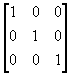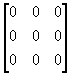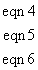Table 5.1 can be transformed to a more convenient form for user input and readability by taking 3 columns at a time for each node. Then, only data for those nodes which are actually constrained is entered. This allows expansion to systems with many degrees of freedom per node without packing too much information on a line. In this spirit, the information contained in table 5.1 can be specified as follows:

( CONSTRAINTS
( NODE 1
( eqn u v w)
(
(1 1 0 0)
(2 0 1 0)
(3 0 0 1)
)
)
( NODE 3
( eqn u v w )
(
(4 1 0 0)
(5 0 1 0)
(6 0 0 1)
)
)
)

Because external constraints are usually defined at the top level only, the multi-level description used in the topology and material specification is unnecessary. Moreover, sparse matrix storage scheme can be used to represent the constraint matrix if it grows too large.

5.5 ENVIRONMENT SPECIFICATION

Environment specification is not yet as well developed as object specification. Attributes of the environment are things such as point and distributed loads, thermal loads, and body forces such as gravity and electromagnetic forces. The environment could also be time and space dependent. The widget subjected to static external point loads serves as a simple environment specification example:

( ENVIRONMENT widget
( node fx fy fz )
(
(1 1.0 1.0 3.0 )
(2 1.0 2.0 3.0 )
(3 1.0 1.0 3.0 )
)
)
)

5.6 ANALYSIS REQUESTS SPECIFICATION

A problem specification could also contain analysis requests, i.e. the kind of analysis the user wants to be performed. Quick changes in the problem are thereby permitted just by changing the specification. Analysis requests for a widget problem might look like:

( WIDGET_ANALYSIS_REQUESTS
( QUANTITIES
displacements
velocities
accelerations
stresses
eigenvalues
)
)

( ACTIVE_PROBLEM_DESCRIPTIONS
( ATTRIBUTE DESCRIPTION ACTIVE OVER TIME )
(
( object widget obj ALL )
( environment widget_1_env 0.0 to 10.0 sec. )
( environment widget_2_env 10.0 to 12.0 sec. )
( methodology widget_how 10.0 to 12.0 sec. )
)
)

5.7 METHODOLOGY SPECIFICATION

Finally, several functions fall exclusively into the category of methodolgy requests. This area is the least developed. Presently, different solution methods are obtained by rewiring the test-bed, i.e. rearranging or coding new routines and recompiling. User specification of the solution methods via the grammar would be nice. Once a good procedure for a family of problems was obtained, it could be saved in a library like any other specification. Possible alternatives are shown in Table 5.2.

 TABLE 5.2 METHODOLOGY ALTERNATIVES Operator Method Solution Or Time Step reduce depth first solution stepping relation constrain breadth first time stepping relation assemble binary region splitting local error tolerance relation reassemble other region splitting local error toleratnce realtion

5.8 TOOLS FOR GRAMMAR IMPLEMENTATION

Once the grammar was designed two powerful software tools were used to generate the code that performs the input language lexical analysis [LESK75] and parsing [JOHN78]. The relationships for the grammar were specified, and this grammar specification was used to generate the parsing program via a compiler-compiler.

The primary job of the parser is to take action based on recognized relationships. A separate program is used to actually recognize the incoming expressions. The program that does this recognition or 'lexical analysis' identifies defined entities known as 'tokens'. An example of a token might be a delimiter such as a left or right parenthesis, or a keyword such as OBJECT, or perhaps TOPOLOGY. Other examples of tokens (also known as terminal symbols) that would be passed to the parser might include a numerical value such as a nodal coordinate, or an object name. When tokens are then passed to the parser, action is taken by the parser to allocate and initialize data structures that contain problem information. The usage of these software tools is discussed in "Yacc: Yet Another Compiler-Compiler" [JOHN78] and "Lex - A Lexical Analyzer Generator" [LESK75].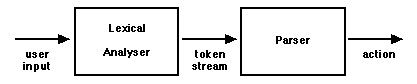FIGURE 5.8 LEXICAL ANALYSER - PARSER INPUTS & OUTPUTS

CHAPTER 6
ILLUSTRATIVE EXAMPLES

The work described herein resulted in a functioning test-bed in which new finite element and substructuring algorithms may be evaluated. Several examples have been run using the test-bed. The recursive substructuring algorithm listed in Chapter 2 was used. The trial element was a bar with 2 degrees of freedom per node. The 'stick plots' of several objects, their object descriptions and their associated frequencies of free vibration are listed below. First we start with a 'piece' object that is made of several bars:

( PROBLEM
( OBJECT piece
( TOPOLOGY
( SUBOBJECTS 4
( subobject name)
(
( 0 bar )  ( 1 bar ) ( 2 bar ) ( 3 bar )
)
)

( NODES 4
(
(0 0.00 0.00 0.00)
(1 0.00 0.50 0.00)
(2 1.00 0.00 0.00)
(3 1.00 0.50 0.00)
)
)

( INCIDENCES 4
( subobj incidences)
(
(0 0 1) (1 0 2)  (2 1 3)  (3 1 2)
)
)
)
)
)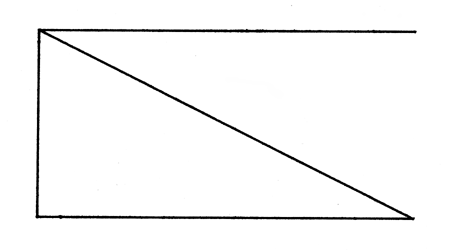FIGURE 6.1 THE 'PIECE' OBJECT

Then we hook these together to form an new object called a 'piece2':

( PROBLEM
( OBJECT piece2
( TOPOLOGY
(NODES 6
(node x y z)
(
(0 0.00 0.00 0.00)
(1 0.00 0.50 0.00)
(2 1.00 0.00 0.00)
(3 1.00 0.50 0.00)
(4 2.00 0.00 0.00)
(5 2.00 0.50 0.00)
)
)
( SUBOBJECTS 2
( subobj name)
(  ( 0 piece)  ( 1 piece) )
)

( INCIDENCES 2
( subobj incidences )
(
( 0 0 1 2 3 )
( 1 2 3 4 5 )
)
)
( BND_NODES 4 (0 1 4 5) ) )
)
)
)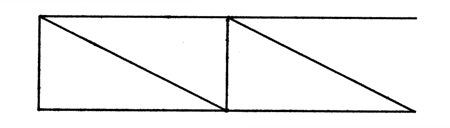FIGURE 6.2 THE 'PIECE2' OBJECT

Then we add these to form an new object called a 'piece4':

( PROBLEM
( OBJECT piece4
(TOPOLOGY
( SUBOBJECTS 2
( subobj name )
(  ( 0 piece2)  ( 1 piece2)  )
)
( NODES 6
( node x y z)
(
( 0 0.00 0.00 0.00)
( 1 0.00 0.50 0.00)
( 2 2.00 0.00 0.00)
( 3 2.00 0.50 0.00)
( 4 4.00 0.00 0.00)
( 5 4.00 0.50 0.00)
)
)
( INCIDENCES 2
( subobj incidences )
(
(0 0 1 2 3)
(1 2 3 4 5)
)
)
( BND_NODES 4  (0 1 4 5) ) )
)
)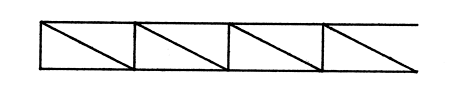FIGURE 6.3 THE 'PIECE4' OBJECT

These are combined to form a 'newbar10':

( PROBLEM
( OBJECT newbar10
( TOPOLOGY
( SUBOBJECTS 7
( subobj name )
(
( 0 bar)  ( 1 bar)  ( 2 piece4)  ( 3 piece4)  ( 4 bar) ( 5 bar)  ( 6 bar)
)
)
( NODES 8
( node x y z )
(
( 0 -1.00 0.25 0.00)
( 1 0.00 0.00 0.00)
( 2 0.00 0.50 0.00)
( 3 4.00 0.00 0.00)
( 4 4.00 0.50 0.00)
( 5 8.00 0.00 0.00)
( 6 8.00 0.50 0.00)
( 7 9.00 0.25 0.00)
)
)
( INCIDENCES 7
( subobj incidences )
(
(0 0 1)
(1 0 2)
(2 1 2 3 4)
(3 3 4 5 6)
(4 5 6)
(5 5 7)
(6 6 7)
)
)
( BND_NODES 2 (0 7) ) )
)
)
)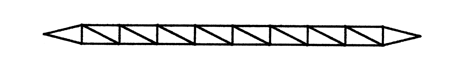5 levels of recursion

FIGURE 6.4 THE 'NEWBAR10' OBJECT

Now before building further we can do an eigenvalue problem and observe the modes of free vibration:w2 = 1.937 x 10-3 s-2

FIGURE 6.5 THE 'NEWBAR10' OBJECT FIRST NONZERO MODE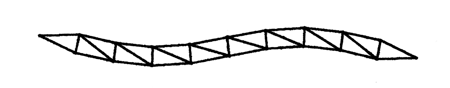w2 = 1.132 x 10-2 s-2
FIGURE 6.6 THE 'NEWBAR10' OBJECT SECOND NONZERO MODE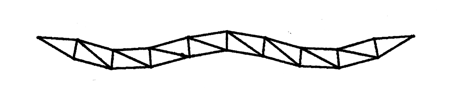w2 = 3.312 x 10-2 s-2

FIGURE 6.7 THE 'NEWBAR10' OBJECT THIRD NONZERO MODE

Having obtained some results for this object, we can now use it to build something new, say for instance a square frame. The objects 'newbar6' and 'newbar8' are similar to 'newbar10' but shorter in length. Their object descriptions are omitted for brevity.

( PROBLEM
( OBJECT bigsquare
( TOPOLOGY
( SUBOBJECTS 5
( subobj name)
(
( 0 newbar6 )
( 1 newbar8 )
( 2 newbar10 )
( 3 newbar8 )
( 4 newbar6 )
)
)
( NODES 4
(
(0 0.00 0.00 0.00)
(1 0.00 6.00 0.00)
(2 8.00 0.00 0.00)
(3 8.00 6.00 0.00)
)
)
( INCIDENCES 5
( subobj incidences)
(
(0 0 1)
(1 0 2)
(2 1 2)
(3 1 3)
(4 2 3)
)
)
)
)
)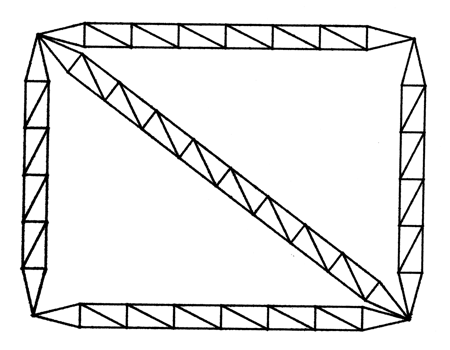6 levels of recursion
FIGURE 6.8 THE 'BIGSQUARE' OBJECTw2 = 3.841 x 10-4s-2
FIGURE 6.9 THE 'BIGSQUARE' OBJECT FIRST NONZERO MODE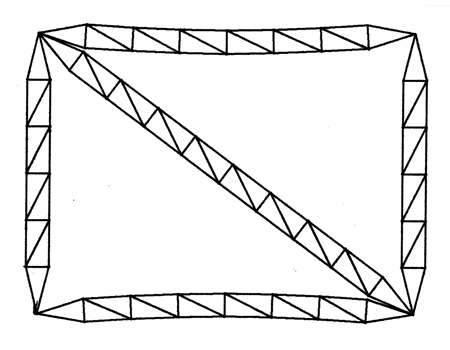w2 = 7.354 x 10-4s-2
FIGURE 6.10 THE 'BIGSQUARE' OBJECT SECOND NONZERO MODE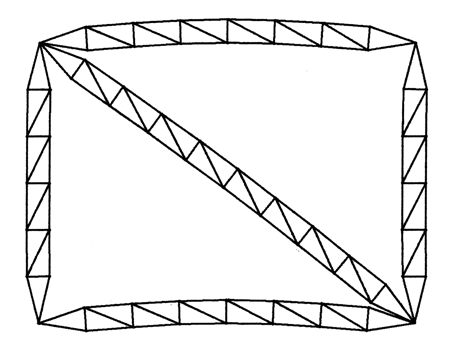w2 = 1.135 x 10-3s-2
FIGURE 6.11 THE 'BIGSQUARE' OBJECT THIRD NONZERO MODE

CHAPTER 7
CONCLUSIONS

Up to now, many computer graphicists have focused on providing realistic looking images as opposed to finite element workers who have focused on generating realistic responding structural system results. The time has come to unify these diciplines so that objects in simulations look and respond realistically. The test-bed development was initiated with these ideas in mind.

The techniques used to develop the test-bed represent a top-down, structured programming approach to

the problem of providing an environment in which new algorithms may be tested. The codes that comprise the test-bed represent a versatile research tool for the evaluation of substructuring and other finite element algorithms as well as the generation of useful data for engineering simulations.

BIBLIOGRAPHY
[BATH82] Bathe, K. J., Finite Element Procedures in Engineering Analysis, Prentice-Hall, Englewood Cliffs, N.J., 1982.

[BENF71] Benfield, W.A. and Hruda, R.F., "Vibration Analysis of Structures by Component Mode Substitution", AIAA Journal, Vol. 9, No. 7, 1971, pp. 1255-1261.

[BERM73] Berman, A., "Vibration Analysis of Structural Systems Using Virtual Substructures", Shock and Vibration Bulletin, Proc. 43, No. 2, 1973, pp. 13-22.

[CHUR41] Church, A. "The Calculi of Lambda Conversion", Annals of Mathematical Studies 6, Princeton University Press 1941, Reprinted by Klaus Reprint Co., 1965

[COHE82] Cohen E., "Subdivision Methods for Curves and Surfaces", Tutorial Notes: Freeform Surfaces, 1982 ACM SIGGRAPH

[COOK81] Cook R.D., Concepts and Applications of Finite Element Analysis, John Wiley & Sons, New York, 1981.

[CRAI77] Craig, R.R. Jr. and Chung, C.J., "Substructure Coupling for Dynamic Analysis and Testing", NASA CR-2781, February 1977.

[DATE76] Date, C. J. Introduction to Database Management Systems, Third Edition Addison-Wesley, 1981, Reading, Mass.

[DODD80] Dodds, R.H. Jr. and Lopez, L.A., "A Generalized Software System for Non-linear Analysis", Advanced Engineering Software, Vol. 2, No. 4, 1980, pp. 161-168.

[DODD82] Dodds, R.H. Jr., Rehak, D.R. and Lopez, L.A., "Development Methodologies for Computationally Oriented Scientific Software", Unpublished Report, 1982.

[DOWE72] Dowell, E.H., "Free Vibration of an Arbitrary Structure in Terms of Component Modes", Journal of Applied Mechanics, Vol. 39, 1972, pp. 727-732.

[FELI81] Felippa, C. A. Architecture of a Distributed Analysis Network for Computational Mechanics Computers & Structures, 1981, Vol. 13, pp. 405-413.

[HALE80] Hale, A.L. and Meirovitch, L., "A General Substructure Synthesis Method for the Dynamic Simulation of Complex Structures", Journal of Sound and Vibration, Vol. 69, No. 2, 1980, pp. 309-326.

[HALE82a] Hale, A.L. and Meirovitch, L., "A Procedure for Improving Discrete Substructure Representation in Dynamic Synthesis", AIAA Journal, Vol. 20, No. 8, 1982, pp. 1128-1136.

[HALE82b] Hale, A.L., "A Family of Subspace Iteration Algorithms for the Eigensolution of Large Structural Systems Composed of Substructures", Proceedings of the First International Modal Analysis Conference, Orlando, Florida, November 8-10, 1982, pp. 500-507.

[HINT75] Hintz, R.M., "Analytical Methods in Component Mode Synthesis", AIAA Journal, Vol. 13, 1975, pp. 1007-1016.

[HURT65] Hurty, W.C., "Dynamic Analysis of Structural Systems Using Component Modes", AIAA Journal, Vol. 3, No. 4, 1965, pp. 678-685.

[JENN77] Jennings, A., Matrix Computation for Engineers and Scientists, John Wiley & Sons, New York, 1977.

[JOHN78] Johnson, S.C., "Yacc: Yet Another Compiler-Compiler", Internal Document, Bell Laboratories, Murray Hill, N.J., 1978.

[LESK75] Lesk, M.E. and Schmidt E., "Lex - A Lexical Analyzer Generator", Internal Document, Bell Laboratories, Murray Hill, N.J., 1975.

[LOPE82] Lopez, L.A., Dodds, R.H. Jr. and Rehak, D.R., "FINITE-Design Concepts, Features and Capability", Unpublished Report, February, 1982.

[MACN71] MacNeal, R.H., "A Hybrid Method of Component Mode Synthesis", Computers and Structures, Vol. 1, 1971, pp. 581-601.

[MEIR80] Meirovitch, L., Computational Methods in Structural Dynamics, Sijthoff-Noordhoff International Publishers, Alphen ann den Rijn, The Netherlands, 1980.

[MEIR81] Meirovitch, L. and Hale, A.L., "On the Substructure Synthesis Method", AIAA Journal, Vol. 19, No. 7, 1981, pp. 940-947.

[NOOR78] Noor, A.K., Kamel, H.A. and Fulton, R.E., "Substructuring Techniques, Status and Projections", Computers and Structures, Vol. 8, 1978, pp. 621-632.

[PARL80] Parlett, B. N., The Symmetric Eigenvalue Problem, Prentice-Hall, Englewood Cliffs, N.J., 1980.

[PETE77] Petersson, H. and Popov, E.P., "Substructuring and Equation System Solutions in Finite Element Analysis", Computers and Structures, Vol. 7, 1977, pp. 197-206.

[PRZE63] Przemieniecki, J.S., "Matrix Structural Analysis of Substructures", AIAA Journal, Vol. 1, 1963, pp. 138-147.

[REYN78] Reynolds, C. "Computer Animation in the World of Actors and Scripts", SM thesis, MIT (Architecture Machine Group), May 1978

[REYN82] Reynolds, C. "Computer Animation with Scripts and Actors", Computer Graphics 1982, Vol. 16, No. 3, pp. 289-296

[SCHR79] Schrem, E., "Trends and Aspects of the Development of Large Finite Element Software Systems", Computers and Structures, Vol. 10, 1979, pp. 419-425.